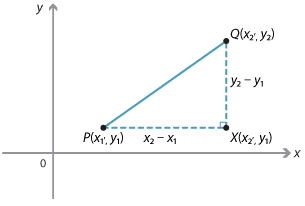Detailed description

We can obtain a formula for the length of any interval. Suppose that $$P(x_1, y_1)$$ and $$Q(x_2, y_2)$$ are two points.

From the right-angled triangle $$PQX$$, where $$X$$ is the point $$(x_2, y_1)$$:

$$PX = x_2 - x_1\ \text{or}\ x_1 - x_2\ \text{and}\ QX = y_2 - y_1\ \text{or}\ y_1 - y_2$$

depending on the positions of P and Q.

By Pythagoras' theorem:

\begin{align}PQ^2 &= PX^2 + QX^2\\\\ &= (x_2 - x_1)^2 + (y_2 - y_1)^2.\end{align}

Therefore $$\hspace{2em}PQ = QP = \sqrt{(x_2 - x_2)^2 + (y_2 - y_1)^2}$$

Note that $$(x_2 - x_1)^2$$ is the same as $$(x_1 - x_2)^2$$ and therefore it doesn't matter whether we go from $$P$$ to $$Q$$ or from $$Q$$ to $$P$$. The result is the same.